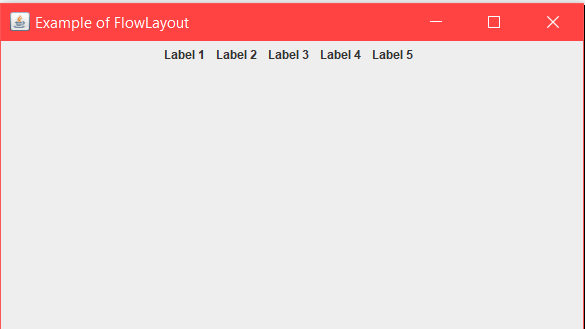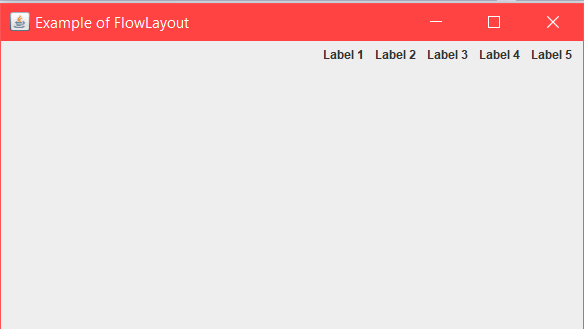# Java AWT | FlowLayout

FlowLayout is used to arrange components in a sequence one after the other. The default layout of applet and panel is FlowLayout.

Constructors :

1. FlowLayout(): It will Construct a new FlowLayout with centered alignment.The horizontal and vertical gap will be 5 pixels.
2. FlowLayout(int align) : It will Construct a new FlowLayout with given alignment.The horizontal and vertical gap will be 5 pixels.
3. FlowLayout(int align, int HorizontalGap, int VerticalGap ): It will Construct a new FlowLayout with given alignment, the given horizontal and vertical gap between the components.
4. JLabel(String text): It will create a JLabel instance with the specified text.

Commonly used methods:

1. setTitle(String Text): This Method is used to set Title of JFrame. The title you want to set is passed as a string.
2. getAlignment(): Returns the alignment for this layout.
3. setAlignment(int align): used to set the alignment for this layout.
4. removeLayoutComponent(Component comp): Used to remove the component passed as argument from the layout.

Below programs will illustrate the Example of FlowLayout in java.

1. Program 1: The following program illustrates the use of FlowLayout by arranging several JLabel components in a JFrame, whose instance class is named as “Example”. We create 5 JLabel components named “l1”, “l2″… “l5” and then add them to the JFrame by the method this.add(). We set the title and bounds of the frame by method setTitle and setBounds.
The layout is set by the method setLayout();

 `// Java program to show Example of FlowLayout. ` `// in java. Importing different Package. ` `import` `java.awt.*; ` `import` `java.awt.event.*; ` `import` `javax.swing.*; ` ` `  `class` `Example ``extends` `JFrame { ` `    ``// Declaration of objects of JLabel class. ` `    ``JLabel l1, l2, l3, l4, l5; ` ` `  `    ``// Constructor of Example class. ` `    ``public` `Example() ` `    ``{ ` `        ``// Creating Object of "FlowLayout" class ` `        ``FlowLayout layout = ``new` `FlowLayout(); ` ` `  `        ``// this Keyword refers to current object. ` `        ``// Function to set Layout of JFrame. ` `        ``this``.setLayout(layout); ` ` `  `        ``// Initialization of object "l1" of JLabel class. ` `        ``l1 = ``new` `JLabel(``"Label 1  "``); ` ` `  `        ``// Initialization of object "l2" of JLabel class. ` `        ``l2 = ``new` `JLabel(``"Label 2  "``); ` ` `  `        ``// Initialization of object "l3" of JLabel class. ` `        ``l3 = ``new` `JLabel(``"Label 3  "``); ` ` `  `        ``// Initialization of object "l4" of JLabel class. ` `        ``l4 = ``new` `JLabel(``"Label 4  "``); ` ` `  `        ``// Initialization of object "l5" of JLabel class. ` `        ``l5 = ``new` `JLabel(``"Label 5  "``); ` ` `  `        ``// this Keyword refers to current object. ` `        ``// Adding Jlabel "l1" on JFrame. ` `        ``this``.add(l1); ` ` `  `        ``// Adding Jlabel "l2" on JFrame. ` `        ``this``.add(l2); ` ` `  `        ``// Adding Jlabel "l3" on JFrame. ` `        ``this``.add(l3); ` ` `  `        ``// Adding Jlabel "l4" on JFrame. ` `        ``this``.add(l4); ` ` `  `        ``// Adding Jlabel "l5" on JFrame. ` `        ``this``.add(l5); ` `    ``} ` `} ` ` `  `class` `MainFrame { ` `    ``// Driver code ` `    ``public` `static` `void` `main(String[] args) ` `    ``{ ` `        ``// Creating Object of Example class. ` `        ``Example f = ``new` `Example(); ` ` `  `        ``// Function to set title of JFrame. ` `        ``f.setTitle(``"Example of FlowLayout"``); ` ` `  `        ``// Function to set Bounds of JFrame. ` `        ``f.setBounds(``200``, ``100``, ``600``, ``400``); ` ` `  `        ``// Function to set visible status of JFrame. ` `        ``f.setVisible(``true``); ` `    ``} ` `} `

Output:We can control the alignment of components in a flow layout arrangement, by using these FlowLayout Fields.
1) RIGHT :- Each row of component shifts towards right.
2) LEFT :- Each row of component shifts towards left.

2. Program 2: The following program illustrates the use of FlowLayout using Right alignment by passing the argument FlowLayout.RIGHT in the constructor of FLowLayout. We create 5 JLabel components named “l1”, “l2″… “l5” and then add them to the JFrame by the method this.add(). We set the title and bounds of the frame by method setTitle and setBounds.
The layout is set by the method setLayout();

 `// Java program to show example of ` `// FlowLayout and using RIGHT alignment ` `import` `java.awt.*; ` `import` `java.awt.event.*; ` `import` `javax.swing.*; ` ` `  `class` `Example ``extends` `JFrame { ` `    ``// Declaration of objects of JLabel class. ` `    ``JLabel l1, l2, l3, l4, l5; ` ` `  `    ``// Constructor of Example class. ` `    ``public` `Example() ` `    ``{ ` `        ``// Creating Object of "FlowLayout" class, passing ` `        ``// RIGHT alignment through constructor. ` `        ``FlowLayout layout = ``new` `FlowLayout(FlowLayout.RIGHT); ` ` `  `        ``// this Keyword refers to current object. ` `        ``// Function to set Layout of JFrame. ` `        ``this``.setLayout(layout); ` ` `  `        ``// Initialization of object "l1" of JLabel class. ` `        ``l1 = ``new` `JLabel(``"Label 1  "``); ` ` `  `        ``// Initialization of object "l2" of JLabel class. ` `        ``l2 = ``new` `JLabel(``"Label 2  "``); ` ` `  `        ``// Initialization of object "l3" of JLabel class. ` `        ``l3 = ``new` `JLabel(``"Label 3  "``); ` ` `  `        ``// Initialization of object "l4" of JLabel class. ` `        ``l4 = ``new` `JLabel(``"Label 4  "``); ` ` `  `        ``// Initialization of object "l5" of JLabel class. ` `        ``l5 = ``new` `JLabel(``"Label 5  "``); ` ` `  `        ``// this Keyword refers to current object. ` `        ``// Adding Jlabel "l1" on JFrame. ` `        ``this``.add(l1); ` ` `  `        ``// Adding Jlabel "l2" on JFrame. ` `        ``this``.add(l2); ` ` `  `        ``// Adding Jlabel "l3" on JFrame. ` `        ``this``.add(l3); ` ` `  `        ``// Adding Jlabel "l4" on JFrame. ` `        ``this``.add(l4); ` ` `  `        ``// Adding Jlabel "l5" on JFrame. ` `        ``this``.add(l5); ` `    ``} ` `} ` ` `  `class` `MainFrame { ` `    ``// Driver code ` `    ``public` `static` `void` `main(String[] args) ` `    ``{ ` `        ``// Creating Object of Example class. ` `        ``Example f = ``new` `Example(); ` ` `  `        ``// Function to set title of JFrame. ` `        ``f.setTitle(``"Example of FlowLayout"``); ` ` `  `        ``// Function to set Bounds of JFrame. ` `        ``f.setBounds(``200``, ``100``, ``600``, ``400``); ` ` `  `        ``// Function to set visible status of JFrame. ` `        ``f.setVisible(``true``); ` `    ``} ` `} `

Output:Don’t stop now and take your learning to the next level. Learn all the important concepts of Data Structures and Algorithms with the help of the most trusted course: DSA Self Paced. Become industry ready at a student-friendly price.

My Personal Notes arrow_drop_upCheck out this Author's contributed articles.

If you like GeeksforGeeks and would like to contribute, you can also write an article using contribute.geeksforgeeks.org or mail your article to contribute@geeksforgeeks.org. See your article appearing on the GeeksforGeeks main page and help other Geeks.

Please Improve this article if you find anything incorrect by clicking on the "Improve Article" button below.

Article Tags :
Practice Tags :

Be the First to upvote.

Please write to us at contribute@geeksforgeeks.org to report any issue with the above content.